Every year, students enter a new math level with new math facts, but what should the students know by the end of each grade? How do teachers measure the success of their students? By using math benchmarks, teachers have a reference point to assess their students’ progress. Rocket Math Online Game and Worksheet Program are great tools to help students meet math benchmarks in every grade.

## What are Math Benchmarks?

Math benchmarks are standard reference points that can be measured and assessed. Teachers use benchmarks in math to help understand where their students are in their math education and know where they need to be in order to succeed in their grade level.

In 1st grade, students focus on learning addition and subtraction up to 20, whole number relationships and grouping, linear measurement and lengths, and geometric shapes.

#### 1. Addition and subtraction up to 20.

1st grade students learn strategies to build math fact fluency for adding and subtracting whole numbers up to 20.

#### 2. Whole number relationships and grouping in tens and ones.

1st grade students learn to compare the whole numbers at least to 100 and be able to understand and solve problems involving their relative sizes.

#### 3. Linear measurement and measuring lengths.

1st grade students learn to understand the meaning and process of measuring including the concepts of iteration and transitivity principle for indirect measurement.

#### 4. Compose and decompose geometric shapes.

1st grade students learn how to compose and decompose geometric shapes, like creating a quadrilateral by putting two triangles together.

In 2nd grade math students focus on developing students’ knowledge of base-ten notation, their fluency with addition and subtraction, standard units of measure, and analyzing shapes.

#### 1. Base-ten system

2nd grade students learn to count by fives, tens and multiples of hundreds, and learn to recognize which digits represent amounts of thousands, hundreds, tens or ones. E.g. 456 is 4 hundreds + 5 tens + 6 ones.

#### 2. Addition and Subtraction up to 1000

2nd grade students develop their math fact fluency in addition and subtraction up to 1000.

#### 3. Standard units of measure

2nd grade students learn standard units of measure and how to use rulers and other measuring tools.

#### 4. Analyze Shapes

2nd grade students learn how to examine shapes by their sides and angles, and decompose and combine them.

In 3rd grade students focus on developing their multiplication and division math facts up to 100, understanding fractions, understanding the structure of rectangular arrays and are, and how to analyze two-dimensional shapes.

#### 1. Multiplication and division math facts up to 100

3rd grade students develop their math fact fluency of multiplication and division of whole numbers up to 100.

#### 2. Fractions and unit fractions

3rd grade students learn unit fractions and fractions and are able to use them to represent numbers equal to, less than, or greater than one.

#### 3. Attribute of two-dimensional regions

3rd grade students learn to find the area of a shape which shows the students how to connect the area to multiplication and how to use multiplication to determine an area of a rectangle.

#### 4. Analyze and compare two-dimensional shapes

3rd grade students compare and classify two-dimensional shapes by their angles and shapes.

In 4th grade, students focus on developing their math fact fluency in multi-digit multiplication and division, learn math operations with fractions, and understanding geometric figures.

#### 1. Multi-digit multiplication & division

4th grade students understand place value to 1,000,000 and know how to apply multi-digit multiplication and multi-digit division to mentally calculate quotients.

#### 2. Fraction equivalence and operations

4thd grade students learn that two fractions can be the same (e.g. 12/16 = 3/4) and learn how to add, subtract, multiply and divide fractions.

#### 3. Geometric figures

4th grade students learn to describe, analyze, compare and classify two-dimensional shapes.

In 5th grade, students focus on developing fraction operations, learn operations with decimals to 100, and develop an understanding of volume.

#### 1. Math fact fluency of fraction operations

5th grade students learn to apply their understanding of fractions and fraction models to calculate sums and differences of fractions in addition, subtraction, multiplication, and division.

#### 2. Relationship between decimals and fractions

5th grade students learn to multiply and divide between decimals and fractions, and to compute quotients of decimals to 100.

#### 3. Volume

5th grade students learn that volume is an attribute of three-dimensional space and can be measured by finding the total number of units that are needed to fill the space.

## Not meeting Benchmarks: what should it mean?

When students score as “not meeting benchmark,” it means the school should provide some intervention to help the students learn.  When it comes to learning math facts, most students have had no opportunity to learn them in a systematic way. Unless they have had an extraordinary school or an unusual teacher, they have not received structured, systematic learning opportunities to memorize math facts. After three decades in schools, I know this to be the case. Without systematic practice and effort, most students will not meet math fact benchmarks. They won’t meet the Common Core expectation that students will “know [math facts] by memory.” Whose fault is that? It is not the student’s fault nor the parent’s fault.

Are you certain your fluency intervention is effective?

The teacher and the school should provide, not just any “intervention” but should provide an effective intervention. Some so-called “interventions” do not reliably produce increased fluency. An essential part of using an intervention is to measure its effectiveness. [That’s why IEP goals are supposed to have measurable short-term objectives!] The best way to measure the effectiveness of a fluency intervention is with timed, curriculum-based fluency assessments.

By measuring the same way for the same amount of time period, you can see if fluency increases. If the student can complete more items during the timed test each time you measure, you can see their fluency increase. When a vast majority of your students, or all of your students, improve in fluency, then you can be certain that the intervention is effective.

## Rocket Math Online Game is effective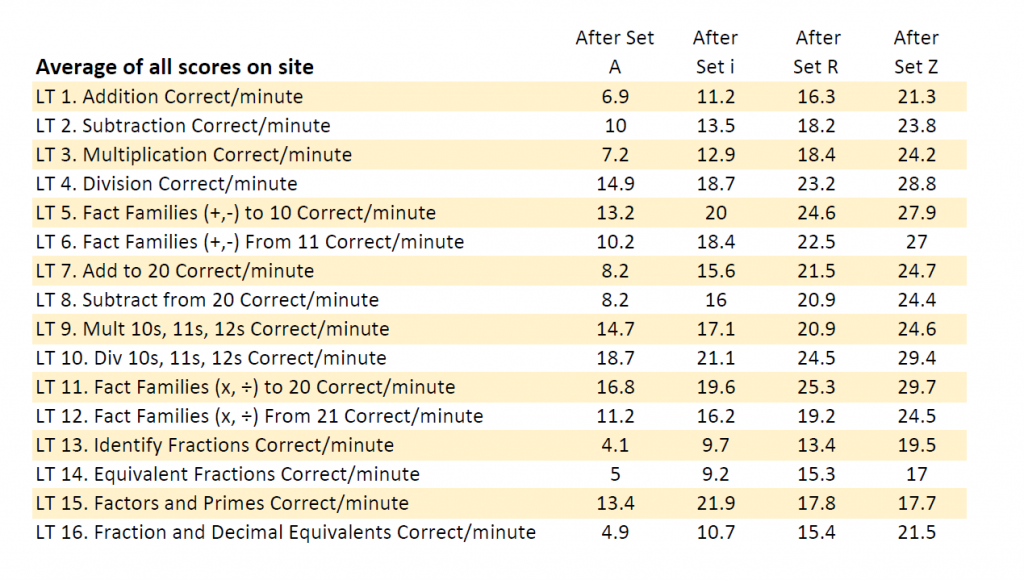As students work through the levels in each Learning Track in the Rocket Math Online Game, they are periodically assigned fluency tests.  Students are tested after completing levels A, i, and R, and then after they finish level Z. Each test is a 1-minute fluency test of a random selection of facts taught in that Learning Track. Therefore the scores are comparable, and when the number goes up at each point in the curriculum, you can be sure that students are increasing in fluency. As you can see from the site-wide data shown here, students consistently improve in fluency as they work through the Rocket Math Online Game. You would not expect students to meet benchmarks until they have completed Set Z in the Learning Track.

## Math fact fluency benchmark in the Online Game: 20/minute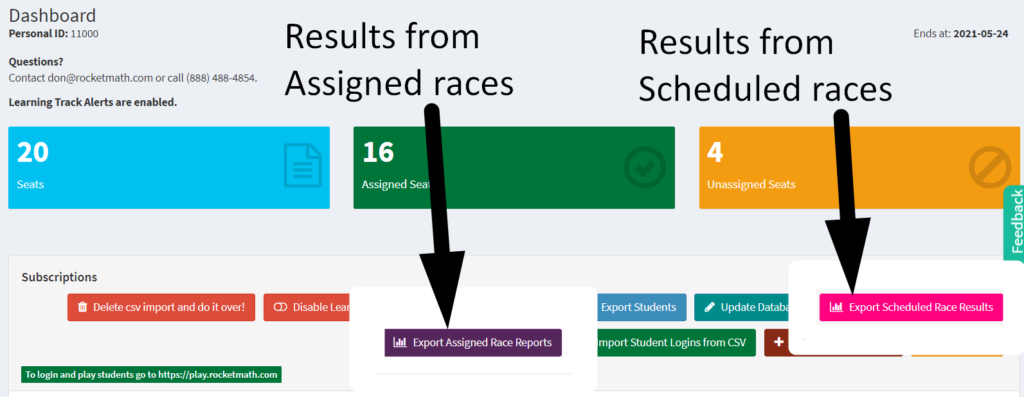The 1-minute RACEs in the Rocket Math Online Game are a good way to measure math fact fluency. You can see from the site-wide averages above that on average, students exceed 20 per minute correct at Set Z, and the average at the beginning is much less. So a reasonable benchmark is 20 correct problems per minute.

The teacher can assign a 1-minute RACE at any time, and the student will need to do it upon their next login. The results will be available in the Review Progress screen and export from the button that gives “Results from Assigned races.” However, the best measure of whether students can meet the benchmark is after Set Z, when they have completed the Learning Track. By clicking on the pink button for exporting “Results from Scheduled races,” the score will appear.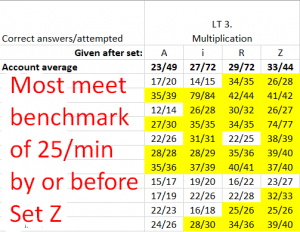To the right is a screenshot of an example of the results from scheduled races from one class of upper elementary students. You can see the Account Average shows improvement at each level. If you set a benchmark at 25, you see all but one of the students met that by the end of Level Z. However, we do see several students who began above that level. (They need not have gone through the Learning Track, but most improved anyway.) After Set Z, those scores show that nearly all students are proficient by the end of the Learning Track. So we know that the Online Game is an effective intervention. But there’s a catch. For this display, we eliminated a lot of students who had not completed Set Z in Multiplication. Students have to play the game to learn.

## Students must participate to learn: Monitor and Recognize Effort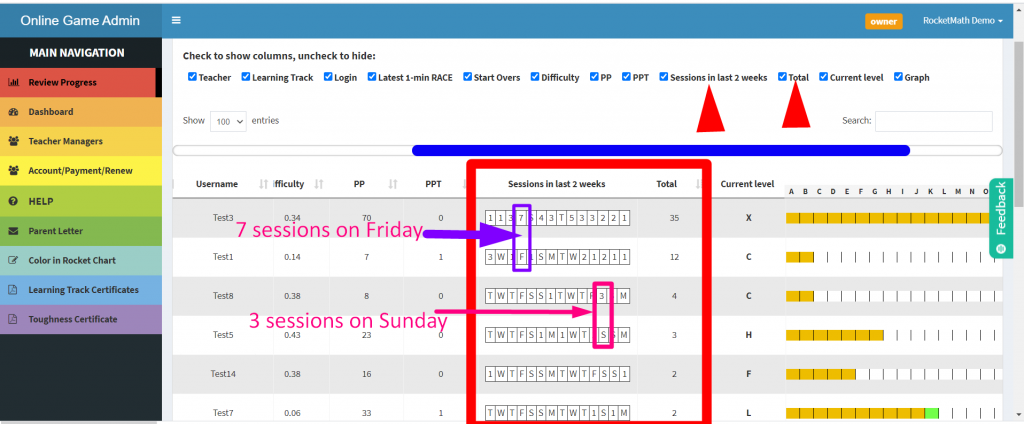Assigning an effective intervention will not help unless students are engaged and participate. Instead of reporting on benchmarks of academic achievement, why not report on benchmarks of effort? Each time students login and complete a session (five, ten, or fifteen minutes in length) on the Online Game, the Review Progress screen will record their session. A mini calendar shows the number of sessions per day. As shown in this screenshot, one student completed 7 sessions on the previous Friday. Another student completed 3 sessions on Sunday. The students below the hard workers, whose mini-calendars showed “F” for Friday and “S” for Sunday, did not complete any sessions because there was no number for those days.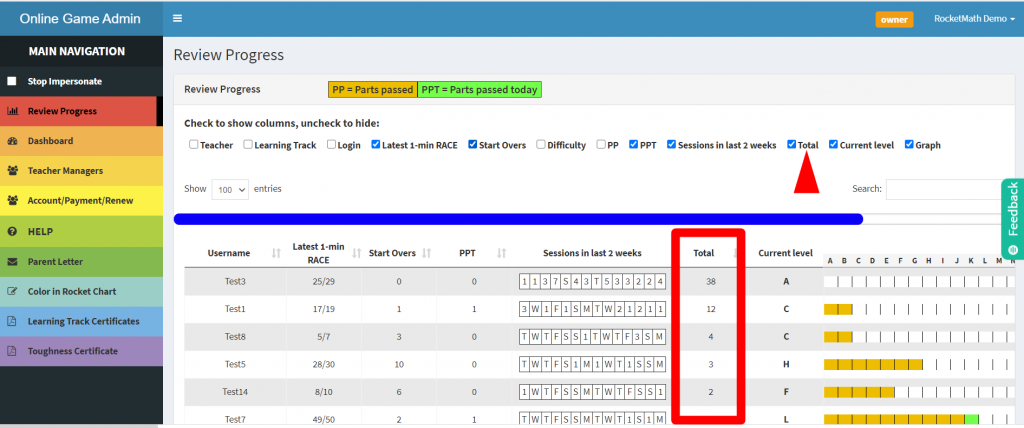Even more discriminative, the Review Progress screen shows the total number of sessions completed in the last two weeks. The picture shows data from our demo accounts. The one for Test3 shows 38 sessions in the last 14 days, while others have only 2 sessions. This total data tells us the level of effort students have been putting forth to learn their math facts. If you monitor this number and recognize students putting forth great effort, you’ll get more students participating. I would say that 10 to 14 sessions in the last two weeks are a good effort. 15 to 24 sessions is a very good effort. Any student with 25 or more sessions in the last two weeks is putting forth great effort and should be recognized as a star!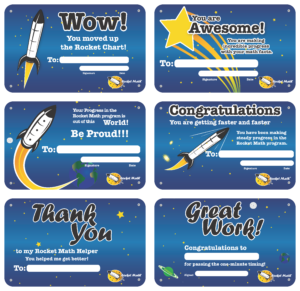You might consider giving out Award Certificates once a month for students who are putting forth super-star effort. If you reward effort, we guarantee you’ll get achievement. We have sets of Award certificates available on our supplements store site. Soon after doing that, you’ll probably have to start awarding Learning Track certificates for students who are completing Learning Tracks. Those are available on the Admin page on the light blue tab in the main rainbow navigation bar.

## Using Rocket Math to Help Students Reach Math Benchmarks

Rocket Math Online Game and Worksheet program will help students reach each grade level math benchmark. By systematically teaching students to be math fact fluency, they will be able to not only succeed but learn to enjoy math.

## Best Math Facts Apps Comparison: Rocket Math vs. XtraMath

There are plenty of math facts apps out there that let students practice math facts they have already learned.  Few apps actually teach math facts.  But apps from Rocket Math and XtraMath are exceptions.  While both apps teach students math facts, one is more effective and fun.

## The two best apps for actually teaching math facts

Math facts apps from Rocket Math and XtraMath effectively teach math facts because they have four essential characteristics:

1. Both math facts apps require students to demonstrate fluency with facts.  Fluency means a student can quickly answer math fact questions from recall.  This is the opposite of letting a student “figure it out” slowly.  Neither app considers a fact mastered until a student can answer a fact consistently within 3 seconds.
2. Both math facts apps zero in on teaching (and bringing to mastery) a small number of facts at a time.  This is the only way to teach math fact fluency. It’s impossible for students to learn and memorize a large number of facts all at once.
3. Both math facts apps are responsive.  Apps simply do not teach if they randomly present facts or do not respond differently when students take a long time to answer a fact.
4. Both math facts apps only allow students to work for a few minutes (15 minutes or less) before taking a break.  Teachers and parents may want to keep students busy practicing math facts for an hour, but students will come to hate the app if they have long sessions.  A few minutes of practice in each session is the best way to learn and to avoid student burnout.
5. Both math facts apps re-teach the fact if a student makes an error.  While both Rocket Math and XtraMath re-teach facts, they re-teach them differently.

While both apps contain these important features and teach math facts, there are a few vital elements that make an effective app like Rocket Math standout.

## An effective app gives a student a sense of accomplishment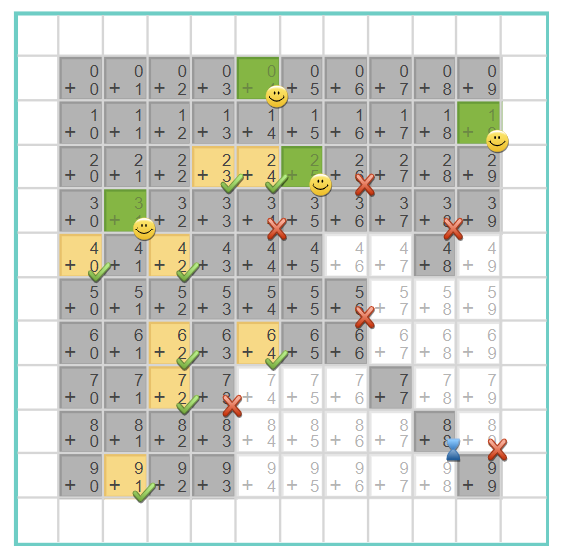The difficult thing about learning math facts is persevering.  There are so many to learn! It takes a while and students have to persevere through boring memorization tasks. The best way to help students learn their math facts is to give them a clear sense of accomplishment as they move through each task.

### How XtraMath monitors progress

To develop a sense of accomplishment among its app users, XtraMath displays math facts on a grid.  XtraMath tests the student and marks the ones that are answered quickly (within 3 seconds) with smiley faces.  It takes a couple of sessions to determine what has been mastered and what hasn’t, so there isn’t a sense of accomplishment at first.  This grid is displayed and explained, but it’s not easy to monitor progress.  Over time, there are fewer squares with facts to learn, but there isn’t clear feedback on what’s being accomplished as students work.

### How Rocket Math monitors progressConversely, Rocket Math begins recognizing student progress immediately and continues to celebrate progress at every step.  The Rocket Math app begins with Set A and progresses up to Set Z.  Each lettered set has three phases: Take-Off, Orbit, and the Universe.  That means there are 78 milestones celebrated in the process of moving from Set A to Set Z.

Take-Off phase has only 4 problems to learn.  They are repeated until the student gets 12 correct in a row.  When the student does that, the doors close (with appropriate sound effects) to show “Mission Accomplished.” They also are congratulated by one of a cast of voices.  Something along the lines of, “Mission Control here.  You did it!  Mission Accomplished! You took off with Set A!  Go for Orbit if you dare!”  With this type of consistent (and fun!) recognition, students clearly understand that they are progressing, and they get the chance to keep learning “if they dare!”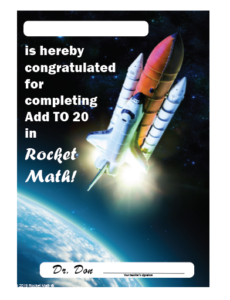In addition to the three phases, students progress through the sets from A to Z.  Each time a student masters a set, by going through all three phases, the student gets congratulated and taken to their rocket picture, as shown above.  When a level is completed, the tile for that level explodes (with appropriate sound effects) and drops off the picture, gradually revealing more of the picture as tiles are demolished.

In the picture above, the tile for “N” has just exploded. After the explosion, a student is congratulated for passing Level N and encouraged to go for Level O if they dare.   When you talk to students about Rocket Math, they always tell you what level they have achieved.  “I’m on Level K!” a student will announce with pride.  That sense of accomplishment is important for them to keep chugging along. Rocket Math also has available Learning Track certificates from Dr. Don and the teacher for completing through Level Z.

## An effective math facts app correct errors—correctly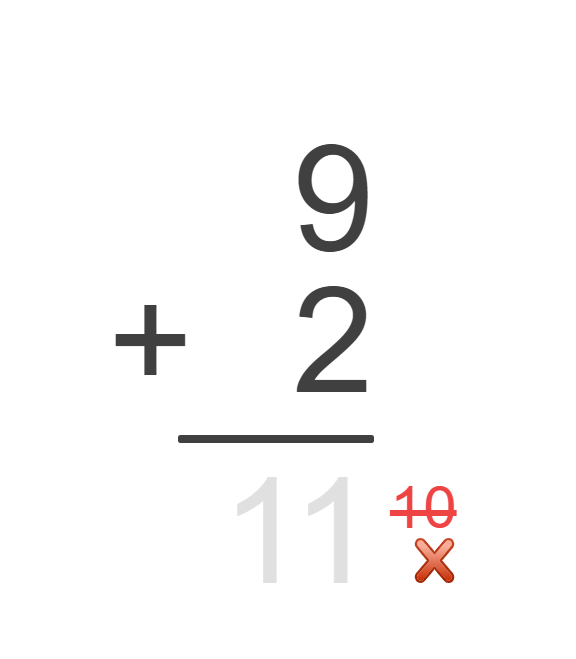Neither of these math fact apps allow errors to go uncorrected.  Students will never learn math facts from an app that does not correct errors.  That puts these two apps head and shoulders above the competition.  However, these two apps correct errors very differently.

### How XtraMath corrects errors

On the left, you can see the XtraMath correction is visual.  If a student enters the wrong answer, the app crosses the incorrect answer out in red and displays the correct answer in gray.  A student then has to enter the correct answer that they see. This is a major mistake. In this case, students don’t have to remember the answer. They just have to enter the numbers in gray.

### How Rocket Math corrects errors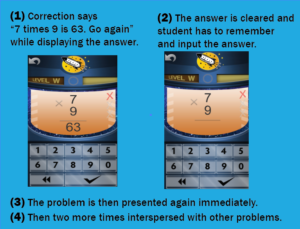Rocket Math, however, requires the student to remember the answer.  When a student answers incorrectly, the screen turns orange and Mission Control displays and recites the correct problem and answer.  In the pictured situation, Mission Control says, “Seven times nine is sixty-three.  Go again.”  Then the answer clears and the game waits for the student to enter the correct answer.  Under these conditions, the student has to listen to the correction and remember the answer, so they can enter it correctly.

Once the student correctly answers that target problem, the app presents the problem again.  Then it presents it twice more interspersed with other problems.

If the student answers the previously missed problem correctly within the three seconds, the game notes the error, and the student continues through the phase.  If the student fails to answer the problem correctly again, the correction process repeats until the student answers correctly.  Having to listen to and remember the answer, rather than just copy the answer, helps students learn better.

## An effective math app gives meaningful feedbackWithout feedback, students can’t learn efficiently and get frustrated. But the feedback cannot be generic. It has to dynamically respond to different student behavior.

### How XtraMath’s app gives feedback

XtraMath’s charming “Mr. C” narrates all of the transitions between parts of each day’s lesson.  He welcomes students, says he is happy to see them, and updates students on their progress.  He gives gentle, generic feedback about how you’re getting better and to remember to try to recall the facts instead of figuring them out.  However, his feedback remains the same no matter how you do.  In short, it is non-contingent feedback, which may not be very meaningful to students.

### How Rocket Math’s app gives feedback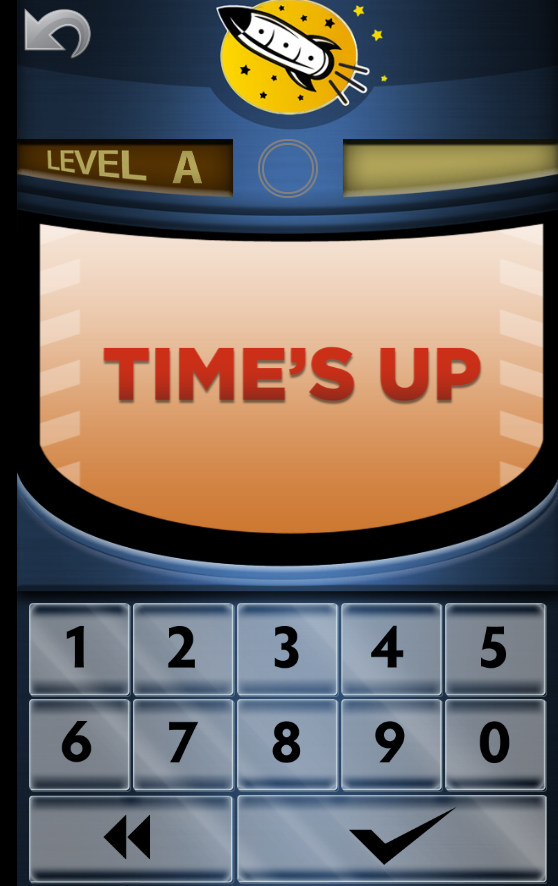Differing from XtraMath, Rocket Math offers students a lot of feedback that is contingent. Contingent feedback means that students will receive different types of feedback depending on their responses.

The Rocket Math app gives positive feedback for all the 78 accomplishments noted above.  It also doles out corrective feedback when the student isn’t doing well.

As noted above, students receive corrective feedback on all errors. They get feedback when they take longer than three seconds to answer too.  The “Time’s Up” screen on the right pops up and Mission Control says, “Ya’ gotta be faster!  Wait.  Listen for the answer.”  And then the problem and the correct answer are given.  Students get a chance to answer that fact again soon and redeem themselves–proving they can answer it in 3 seconds.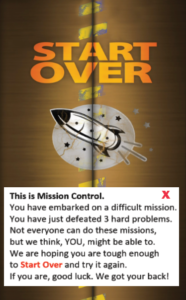The app tracks errors and three errors means the student needs more practice on this part.  The doors close (with appropriate sound effects).   The student is given encouragement that they have defeated three hard problems, and a challenge to Start Over if they are tough enough.  At that point, the “go” screen appears and the student has to hit “go” to open the doors (with appropriate sound effects) and try it again.

When it comes to recognizing a student’s success, the Rocket Math app holds nothing back. After a student completes a phase, one of a cast of voices gives enthusiastic congratulations as noted above.

Typically, students don’t have to “start over” more than once or twice in a phase, but they still feel a real sense of accomplishment when they do complete the phase.  The feedback students get from Rocket Math matters because they have to work hard to earn it.

## How much does an effective math facts app cost?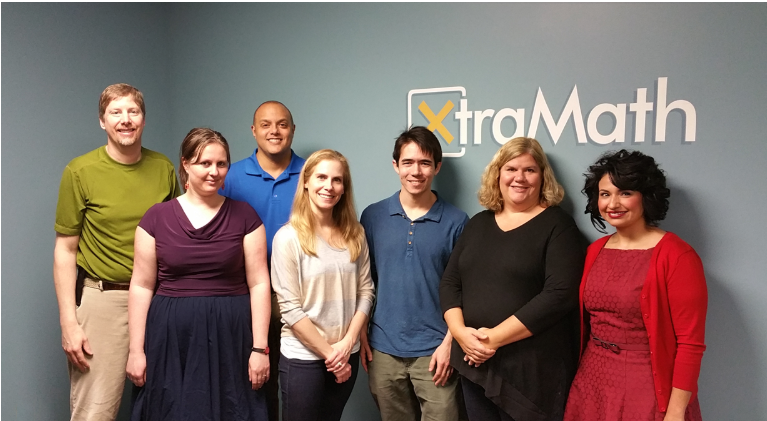It is hard to beat the price of XtraMath, which is free.  XtraMath is run by a non-profit based in Seattle.  They have a staff of six folks in Seattle, and they do accept donations.  Their product is great, and they are able to give it away.Rocket Math is run by one person, Dr. Don.  He supports the app, its development and himself with the proceeds.  He answers his own phone and is happy to talk with teachers about math facts.  The Rocket Math Online Game is a good value at \$1.15 a year per seat (when ordering 100 or more seats).  Twenty to 50 seats are \$2.30 each. And fewer than 20 seats cost \$4 each per year.  As one principal-customer of Rocket Math said, “We used to have XtraMath.  We’d rather pay a little bit for Rocket Math because the kids like it better.”

# Rocket Math Wins Editors Choice Award From All Digital SchoolRocket Math is pleased to announce that we have won the editor’s choice award in math programs from All Digital School (ADS). With so many turning to online education, Rocket Math has been uniquely positioned to grow and adapt to these changing times. Our program has helped thousands of students across the country to learn math efficiently and enjoyably. We’re glad that this hard work has paid off and are thrilled to be recognized for our efforts.

All Digital School is a digital educational resource platform that compiles educational materials and tools that students everywhere can use online. ADS was founded to help educators, parents, and students to find the best materials, websites, and tools available during the pandemic. Their goal is to help everyone receive the best possible learning experience, no matter where they live. ADS is committed to providing an accessible online community for teachers and parents, creating a space where fellow educators can compare notes and review resources. This valuable online education provider now has over 4000 active resource listings for students of all ages.

You can see the Rocket Math program page at ADS here.

Editors at ADS regularly review their listings and choose resources that stand out in their respective fields. In the most recent review of mathematics programs, Rocket Math was selected as a standout favorite and recognized with an editor’s choice award. This distinctive honor pushes us to work even harder and demonstrates our unwavering commitment to provide every student with the opportunity to master math facts.

About Rocket Math:  For over a decade, Rocket Math has served as an educational resource that allows students to learn math quickly, thoroughly, and happily. Rocket Math stresses that all students can learn math and that students are motivated by seeing their own progress and success.

## Teach Multiplication Facts to Struggling Pre-Algebra Students

You want your middle-grade students to complete the pre-algebra math topics so they are ready to begin to study algebra in 8th or 9th grade. A disheartening number of middle-grade students have not memorized basic multiplication facts (times tables). Students must know multiplication facts to follow, absorb, and implement pre-algebra topics.  How to teach multiplication facts to struggling students? How can a teacher help their struggling students learn multiplication facts when a lot of their students do not need to do that work?

Rocket Math Online Game includes learning tracks for pre-algebra skills as well as basic multiplication and division facts. Within the Rocket Math Online Game, teachers assign students the learning tracks that they most need.

## Math Strategies for Struggling StudentsStudents who do not know their multiplication facts are constantly distracted from learning math strategies by having to stop and “figure out” basic facts. Every time they are asked to provide the answer to a multiplication fact, they have to turn their attention to working it out or looking it up. By the time they have gone through their process, they have lost the thread of the strategy they are supposed to be learning. The most important thing a math teacher can do for struggling math students is to help them bring math facts to automaticity. Then answering math fact questions no longer interferes with learning multi-step strategies for solving math problems.

## Why Multiplication facts are Important to Learn Before Middle School MathMany pre-algebra math topics assume students have a ready knowledge of multiplication facts to even understand. When I was a middle-grade teacher, my remedial students were unable to follow or understand topics such as Finding factor pairs, reducing fractions, equivalent fractions, converting fractions, unlike fractions, and so on. I realized that it was because they did not know basic multiplication facts. When I reduced 8/24 to ⅓ it was like magic because they did not quickly recognize the multiplication facts involved. They didn’t understand the concepts we were trying to learn because they did not see the relationships they were supposed to know. When I asked them to think of the factor pairs of 36 they were unable to find them all, no matter how much time I gave them. While students can do multi-digit multiplication problems using a times table chart, it does them no good in pre-algebra topics because it takes too long, even if they know what to look up. Now let’s look at how to teach multiplication to struggling students using Rocket Math Worksheets or Rocket Math Online Game.

## How to Teach Multiplication to Struggling Students Using Rocket MathStudents who have not yet mastered multiplication facts are going to require a very effective teaching methodology to learn them. The haphazard, leave-it-up-to-the-student methods have already failed them. By now, these students lack confidence in their ability to learn the facts, so you need a sure-fire system.

Rocket Math is just such a system. Both the Worksheet Program and the Online Game systematically introduce students to the facts in a careful sequence that they can do. The Worksheet Program and Online Game ask students to memorize only two facts and their reverses at a time.

Students demonstrate mastery of those facts by answering them without hesitation. Then Rocket Math will add two more facts and their reverses. Small steps at a time, systematically the students can memorize the facts and answer them instantly from memory. If students practice every day, within a few weeks you’ll see a dramatic improvement in their recall of multiplication facts.

But what about the students who already know their multiplication facts? Rocket Math has something for them as well.

## Rocket Math Programs for Advanced Students

Teachers can assign Rocket Math as a 10-minute warm-up or cool down for all their students whether they are behind or advanced. Rocket Math has several pre-algebra topics for those students who already know their multiplication facts. Each of these topics will help them do pre-algebra processes more fluently and to quickly recognize relationships that they have memorized.

### Learning Track 13: Identifying Fractions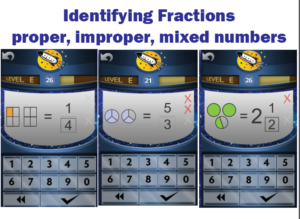When students initially learn about fractions they are often only shown proper fractions. As a result, they have a limited understanding of fractions and can be confused by improper fractions or mixed numbers. The Rocket Math programs (both Worksheet and Online) prevent this problem.  From the start, we teach students using examples of both proper and improper fractions as well as whole numbers and mixed numbers. Students learn to identify over 90 different fractions quickly and easily by getting lots of practice. Their understanding of fractions will deepen and become more flexible as they learn to recognize many examples of fractions.

### Learning Track 14: Equivalent Fractions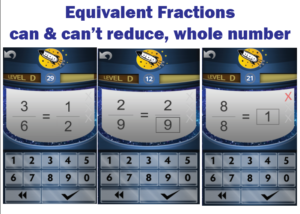Students will memorize the most common equivalent fractions with this Rocket Math Learning Track. They will also learn to identify a number of fractions, such as 2/9,  that do not “reduce” or for which there are no equivalent fractions in lower terms. Students also learn to recognize a fraction equal to 1 whole in its various forms. When students don’t instantly know the answer they are told the equivalent fraction and given practice on it. The computer gives help in the Online Game.  Their partner gives that help in the Worksheet Program. By the end of the program, students will learn over 90 equivalent fractions. This gives students an excellent start on being able to manipulate fractions quickly and easily.

### Learning Track 15: Factors & Primes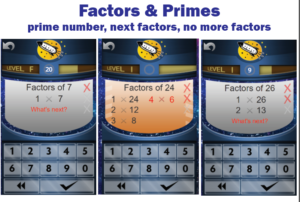Students are required to “find the factors” when dealing with unlike fractions and reducing fractions. Rocket Math Worksheet and Online Game teach students how to find factor pairs. Students learn how to find all the factor pairs and what they all are for many common numbers. They also learn to identify prime numbers and their characteristic of having only one and themselves as factors. Students learn the factor pairs in order and know the “last” factor pair when they see it. When the game asks “What’s next?” students can provide the next pair of factors or click the checkmark to indicate there are no more factors. When students go through this Learning Track they will no longer hesitate when asked for the factors of common numbers.

### Learning Track 16: Fraction & Decimal Equivalents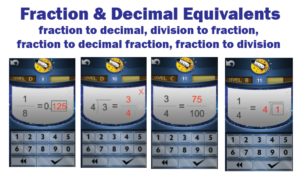Common fraction and decimal equivalents should not require a laborious process to “figure out.” Students should just know these, so this Learning Track in the Online Game allows them to memorize a bunch of common decimal and fraction equivalents. Having a facility with a lot of fraction and decimal equivalents means faster computation as well as a way to check their process when manipulating fractions and decimals. Students also learn another essential pre-algebra skill that often confuses them.  They learn to correctly and fluently translate a fraction into a division problem and vice-versa.

“Test Drive” any of the 16 Learning Tracks in our Online Game demo accounts.

## What are Math Drills?

Math drills are exercises given to students that can help improve their speed and ease of recall. The goal of math drills is to help students develop automaticity, allowing them to instantly recall from memory the answer to any math fact. If carefully designed timed math drills can help check which facts the student has learned and which ones the student needs to work on. If not carefully designed, they can be a terror to children. Carefully designed math drills in the elementary grades can smooth the way for easy success later on in math.

###Why Is Math Fact Fluency Important?

Math fact fluency is important because it is the first step to developing automaticity. Automaticity frees up the students’ short term memory for more important questions. It means students can answer basic math facts like 7 x 9 or 4 + 8 instantly, by recall without effort. Students who aren’t fluent in math facts, have to stop and figure out facts, and then won’t be able to focus on higher-order math lessons. This could lead to them missing parts of the instruction.

## How Do Online Math Drills Help Children Develop Math Fact Fluency?

Correctly recalling the answer to a math fact strengthens the neural connection between the problem such as 9 plus 7, and its answer, 16. [Note that repeating a fact over and over does not achieve the same result. Finding the answer in memory and producing it is what strengthens that connection.] Math drills that ask students to recall answers to a couple of targeted facts, mixed in with other facts the student already knows, makes those neural connections stronger until they can answer those targeted facts correctly, and eventually without any conscious thought. The curriculum should not go on to target any more new facts to learn until the student is fluent with the ones learned so far. A computer program is able to patiently provide this practice for as long as each student needs, which is wonderful.

### Why are Math Tests Timed?

Math tests are timed to tell if students are solving math facts by recall rather than deriving the answer. By timing the tests, teachers can tell which students are able to recall answers instantly and which ones need more help to develop automaticity.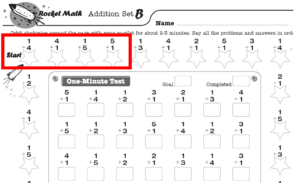Before I understood this, I made students do pages of mixed math facts, which they did by figuring them out.  However, that practice was not helping them become fluent. Timing those pages of mixed facts would not have helped either. In graduate school, I was taught the learning principles that would help students develop fluency. Students need to focus on a small number of facts so they can recall them. So math drills should be composed of a carefully selected set of facts.  This is the key to the design of Rocket Math and is why it works so well.

## What Kind Of Drills Should Your Child Do?

At the very least, your child should learn the basic 1s through 9s math facts of addition, subtraction, multiplication, and division. These will make a huge difference in your child’s success in math. However, if you want to help your child become really proficient and confident in math, there are more things you can drill at each grade level.

###Kindergarten Math Drills

Kindergarteners need to be able to count objects as well as rote count. They should practice counting up to 20, at least, and counting by tens to 100. They should learn how to write the numerals and be given drills to practice numeral formation. Obviously this is not something that can be practiced online, but a good writing practice program such as Rocket Writing for Numerals will set your child up for success.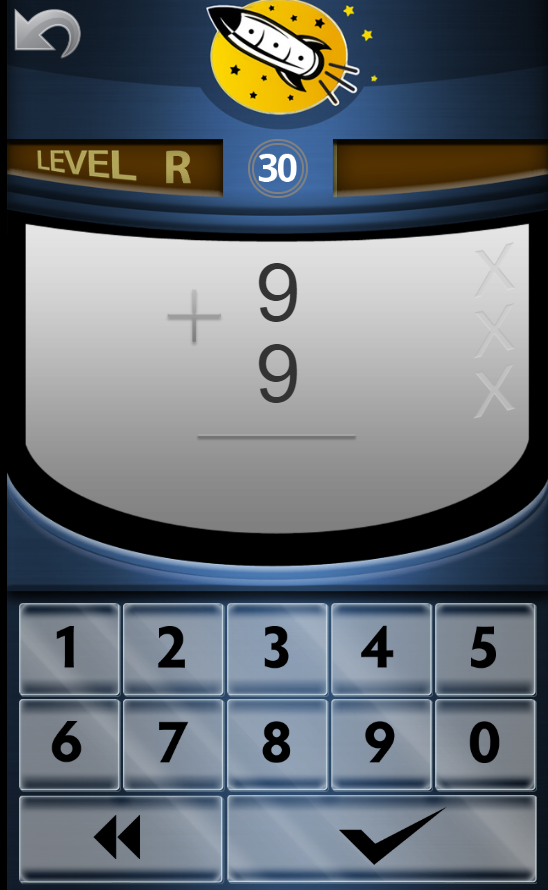In first-grade, learning how to count and write numerals is assumed. The key skill to drill on in first-grade is addition facts 1 through 9. Once those are learned, children can drill on addition facts to 20, such as 13+6, or 4+15. You can also drill first-grade students on fact families, which combine addition and subtraction facts. A fact family example is 3+2, 2+3, 5-2, 5-3. In first-grade fact families (+, -) up to 10 is a reasonable amount to learn.

If addition skills from first-grade are mastered, then drilling on subtraction facts 1s through 9s are the top priority. Once those are learned, students will benefit from drilling up to the 20s in subtraction, such as 17-5 or 19-8. You can also drill second-grade students on fact families, which combine addition and subtraction facts. In second-grade, once fact families up to 10 are mastered, you can drill them on fact families from 11, such as 8+5, 5+8, 13-5, 13-8. Skip counting, or counting by a number (such as by fours- 4, 8, 12, 16, 20, 24, 28, 32, 36, 40), is useful to learn in second-grade. Also, second-grade is not too soon to begin drilling students on identifying fractions.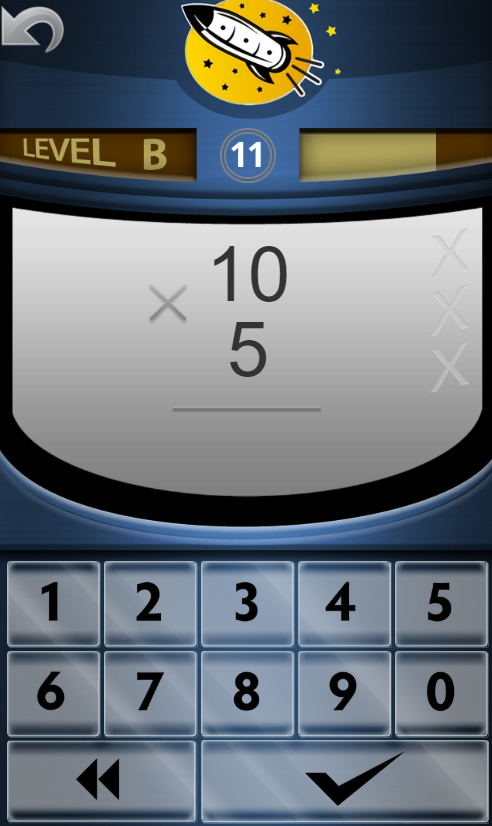Starting in third-grade multiplication facts is essential from this point onward and can’t be counted on fingers. The basic multiplication facts 1 through 9 must be memorized and drills are the only way to do that. Even if addition and subtraction are not yet mastered it is essential to get multiplication facts learned. Then you can go back and pick up addition and subtraction.

Alternatively, or additionally, students can begin drilling on fact families in multiplication and division. An example of this kind of fact family is 4 x5 , 5 x 4, 20 ÷ 4, 20 ÷ 5. Fact families up to 20 are enough in third-grade.

After multiplication facts 1 through 9, you can move on to drilling the 10s-11s-12s. Also, Identifying Fractions is something that third-grade students can become fluent in. Factors (finding all the factors of a number) can be drilled at this grade level or any time in the next three years.

In fourth-grade, it is essential that multiplication facts 1s through 9s are in place first before drilling on division facts. Students will quickly realize division facts are just the opposite of multiplication facts. Once division facts 1s through 9s are learned, you should go back and make sure that addition and subtraction are mastered. After addition and subtraction facts are automatic, then start students on multiplication 10s-11s-12s and division 10s-11s-12s. Once all of these are mastered, students can work on either identifying fractions or factors. Another skill that can greatly help students in later grades is memorizing equivalent fractions.Students in fifth-grade or higher should be fluent in all these basic math areas: multiplication (1s through 9s), addition (1s through 9s), subtraction (1s through 9s), and division (1s through 9s). These can be strengthened by doing fact families with each of these operations: fact families (+, -) to 10, fact families (+, -) from 11, fact families (x, ÷) to 20, and fact families (x, ÷) from 21. Once these are done, students can start drilling on factors, identifying fractions, equivalent fractions, then learning to add and subtract integers. Then the students can start multiplication 10s-11s-12s and division 10s-11s-12s. The same sequence applies in any grade after fifth. The more facts learned in math to the level of automaticity, the easier the rest of math will be.

The Rocket Math Online Game provides the right amount of drill to help your child learn these basic skills. Providing plenty of practice, it is timed and requires students to recall facts (answering within 3 seconds) before moving on to learn more facts. They work their way up through 26 sets, from Set A to Set Z, learning more facts as they go along. The game provides many milestones of progress, lots of little breaks and congratulations as students progress through the twelve Learning Tracks. You can place your child in these tracks in the order you choose. The Rocket Math Online Game includes the following twelve Learning Tracks.

2. Subtraction 1s through 9s
3. Multiplication 1s through 9s
4. Division 1s through 9s
5. Fact Families (+, -) to 10, ex.4+6, 6+4, 10-4, 10-6
6. Fact Families (+, -) from 11, ex. 5+6, 6+5, 11-6, 11-5.
7. Add to 20, example 13+4, 4+13,
8. Subtract from 20, example 15-3, 15-12,
9. Multiplication 10s-11s-12s,
10. Division 10s-11s-12s.
11. Fact Families (x, ÷) to 20, example 4×5, 5×4, 20÷4, 20÷5
12. Fact Families (x, ÷) from 21, example 3×7, 7×3, 21÷3, 21÷7

## The Rocket Math Worksheet Program also provides math drills

Math drills are important for setting your students up for success later in life. Help them build their automaticity and become fluent in the basic math facts through Rocket Math Worksheet Program. The Rocket Math Worksheet Program includes more Learning tracks than the Online Games and you can use this program to help your students with basic math skills.

Here is a list of the worksheets in the Rocket Math Worksheet Program.

## Math Fact Benchmarks: Why Writing Speed Matters

Math drills are used to help students learn their basic single-digit math facts. The goal is to help students answer basic math facts from recall and develop automaticity. The only way to tell if a student is recalling the answer rather than figuring it out is by using timed math drills as benchmarks. But students cannot learn how to recall a full page of math facts by drilling on them. The drills will likely cause anxiety and frustration for students. Here are some common pitfalls that are wise to avoid when teaching students basic single-digit facts.

## Common Problems With Math Benchmarks

Schools typically set “benchmarks” to evaluate whether students have achieved their educational goals.  When it comes to math fact memorization, schools will set benchmarks to distinguish between knowing the answers by a quick recall from the slow process of figuring out the facts. There are two main problems with these benchmarks.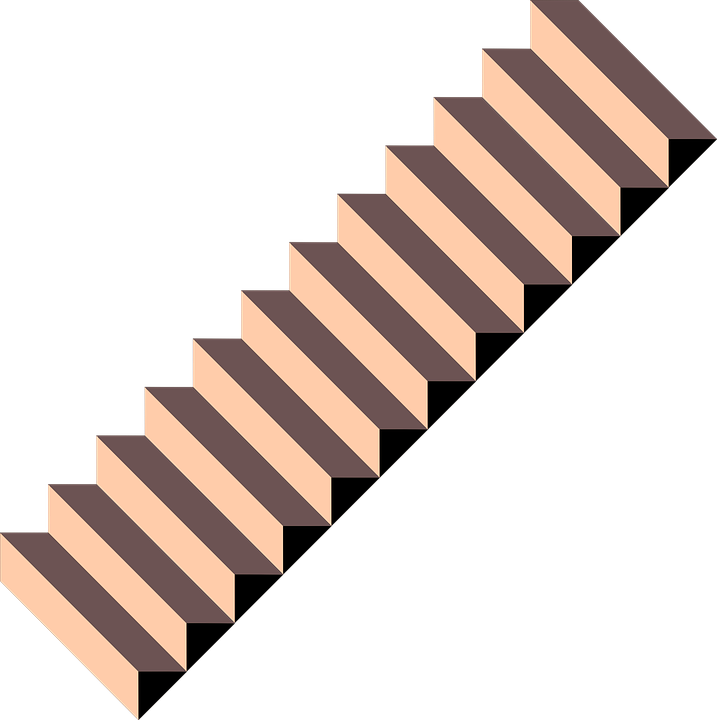### Learned one at a time.

The first problem is that math facts are learned individually and students meet the benchmark one problem at a time. The benchmark should be to answer a problem in less than a second. If they can, then it would be clear which math facts a student has memorized and which ones they don’t. It is more common for schools to give tests on all the facts in an operation and these tests can’t tell the schools which facts are memorized. A more meaningful report would be which facts in an operation can a student answer instantly.### Time to write the answer.

The second main problem is that no matter how well students know facts, they cannot write answers to facts any faster than they can write. Elementary students vary in speed and can write answers anywhere from 10 answers in a minute to 50 in a minute. If a student can answer 40 problems in a minute, they have achieved mastery of math facts. But if they can’t write answers that quickly, they can not meet their school’s benchmark. In order to set a reasonable standard, teachers need to know how fast students can write and a writing speed test will help determine this.

## Common Core Math Fact Fluency Need Not Cause Anguish

The Common Core Idea says that students must have a quick recall of math facts to progress successfully in math. For some reason, almost all children have memorized 2+2=4 and can answer this problem from recall. It is not stressful or hard and will not cause math phobia. So, we know that learning how to answer a math problem instantly from recall is doable and can be done without being stressful. The challenge is that there are a lot of facts to learn, but taken a few at a time, they can all be learned equally easily.

To do this though, it takes a systematic effort, daily practice, and careful monitoring. Teachers need a facts program of some kind to help their students commit a large number of facts to memory. Before developing the design principles behind Rocket Math, I had thought this was a nearly impossible task. But in the last 20 years, I have seen how Rocket Math has been used successfully to teach math facts to students. With plenty of time, patience, and encouragement, students can learn to recall math facts from memory and with Rocket Math they can do this while having fun.

## Rocket Math Writing Speed Test

Here is a simple easy-to-use Writing Speed Test to help figure out how fast a student is able to answer when they have all the facts in an operation memorized. The test is a mix of one and two-digit numbers so it works with addition and multiplication.

Simply give the test to your students and have them write the numbers they see in each box for one minute. You will be able to find out how many boxes they can complete in one minute. That number is the upper limit of math fact problems you can expect them to be able to answer–if they are answering from recall rather than by figuring them out.

### Writing speed is number of boxes completed.

When the student has finished the test, you will have the number of boxes they can complete in a minute. You can expect the student to be able to finish at least 80% of that number if they can recall the facts instantly.  If the student can answer 90%, they don’t need any fact work. If they are between 80 and 90% of that number, they are good, but more facts work would help them. Anything below 80% and they are having to stop to figure out some of the facts and need more work.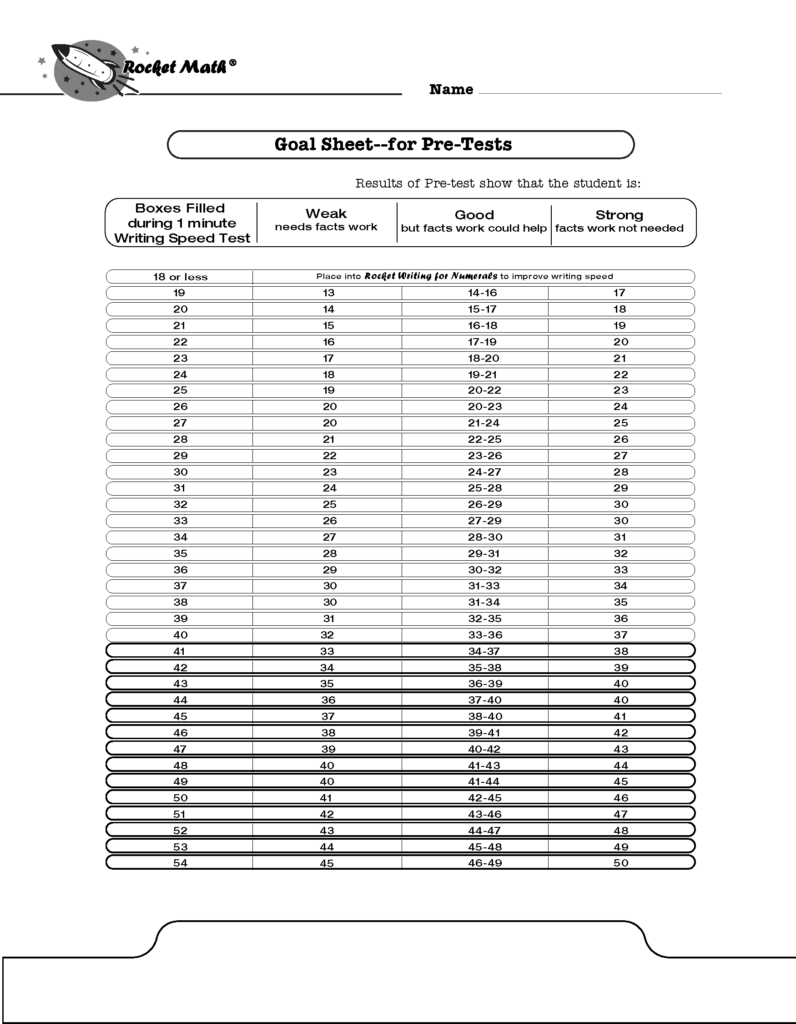If you’re not interested in doing the math over and over, here is a goal sheet for pre-tests, where the numbers are worked out for you. You can print it from this link.

### Benchmarks must be based on writing speed.

Benchmarks that don’t take into account the speed at which children can write, leave a lot of children with an impossible expectation. Asking children to do something they are physically incapable of doing will cause a lot of unnecessary anguish.

## Kindergarten Math BenchmarksStudents should be taught how to write numerals correctly and efficiently in kindergarten. The methods of drawing numerals that children invent on their own can be slow, cumbersome, and inefficient – causing them to have a slow writing speed later. Rocket Math Writing for Numerals is a systematic method that will help students learn how to form their numerals. Benchmarks for kindergarteners should focus on the speed of writing numerals, which takes a good amount of practice and instruction.

Kindergarten Numeral Writing Fluency Benchmarks (digits)

• Mid-year
• 20 digits per minute
• End of year
• 40 digits /minuteFirst-grade students need to be fluent in writing numerals. Not every kindergarten does this, so teachers should test and give some kind of numeral writing program to students who do not meet these writing standards.

First Grade Numeral Writing Fluency Benchmarks (digits)

• Start of year
• 40 digits per minute
•  Mid-year
• 60 digits per minute
•  End of year
• 60 digits per minute

We also want first-graders to learn addition facts to the level of instant recall. The student’s writing speed is the number of boxes they can complete in one minute.

First Grade Math Fact Fluency Benchmarks (problems)

• Start of year
• Addition 20% of writing speed
•  Mid-year
• Addition 40% of writing speed
• End of year
• Addition 80% of writing speed
• Subtraction 20% of writing speed

In second-grade we want students to master subtraction facts as well as addition.

• Start of year
• Addition 80% of writing speed
• Mid-year
• Addition 80% of writing speed
• Subtraction 40% of writing speed
• End of Year
• Addition 80% of writing speed
• Subtraction 80% of writing speedIn third-grade it is important that students master subtraction and begin working on multiplication.

• Start of year
• Addition 80% of writing speed
• Subtraction 80% of writing speed
• Mid-year
• Addition 80% of writing speed
• Subtraction 80% of writing speed
• Multiplication 40% of writing speed
• End of year
• Addition 80% of writing speed
• Subtraction 80% of writing speed
• Multiplication 80% of writing speed

In fourth-grade it is important for the students to have multiplication mastered and begin division.

• Start of the Year
• Addition 80% of writing speed
• Subtraction 80% of writing speed
• Multiplication 80% of writing speed
• Mid-year
• Addition 80% of writing speed
• Subtraction 80% of writing speed
• Multiplication 80% of writing speed
• Division 40% of writing speed
• End of year
• Addition 80% of writing speed
• Subtraction 80% of writing speed
• Multiplication 80% of writing speed
• Division 80% of writing speed

## Fifth-grade (and up) math fact benchmarksIn fifth-grade it is important for the students to have mastered their basic math facts in addition, subtraction, multiplication, and division.

• Start of year
• Addition 80% of writing speed
• Subtraction 80% of writing speed
• Multiplication 80% of writing speed
• Division 80% of writing speed
•
•
• Mid-year
•  Addition 80% of writing speed
• Subtraction 80% of writing speed
• Multiplication 80% of writing speed
• Division 80% of writing speed
• End of year
• Addition 80% of writing speed
• Subtraction 80% of writing speed
• Multiplication 80% of writing speed
• Division 80% of writing speed

All the 1-minute pre-tests and the writing speed tests and the goal sheet can be found on this page https://rocketmath.com/pre-tests/.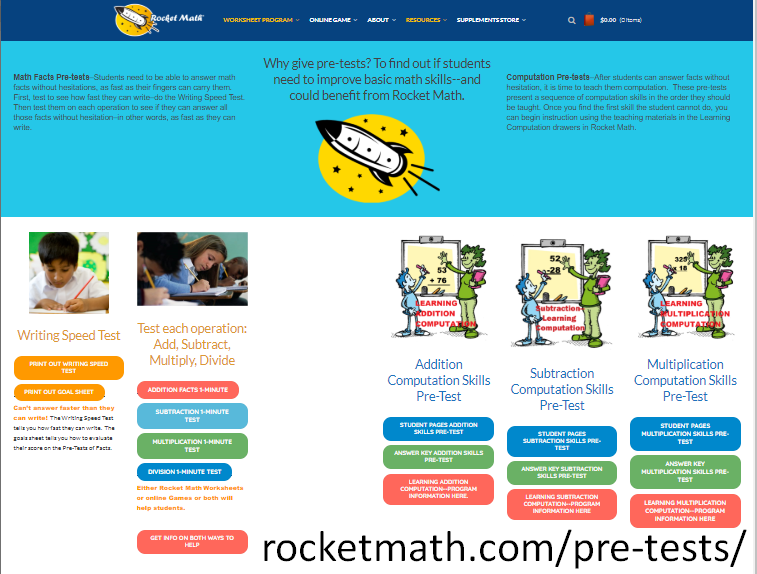## Rocket Math Is A Fun Way For Students To Learn

Rocket Math has a systematic approach to teaching students how to become fluent in basic math facts and still have fun learning math. Math can seem daunting to learn and if not taught properly, using a good math program, students can build a math phobia. Teachers have been using Rocket Math for over 20 years now and believe it to be an invaluable tool to help their students learn math.

## Rocket Math Teaches Math Facts Fast!

Do your students struggle to complete their timed math worksheets? Is your classroom a sea of finger-counting during fast math facts practice?

Your students aren’t the problem. It’s your teaching technique that’s hindering progress.

Help your students learn math facts quickly and gain confidence in their skills with Rocket Math’s research-based program. The program works because it teaches memorization through multiple, evidence-based techniques that work for all types of learners.

## Why Faster Is BetterLearning math facts at a young age plays a key role in a student’s ability to succeed throughout their education as well as out in the real world.

Not only does math fact memorization serve as a foundation to other math skills, but it also plays a part in motivating student. Most children will feel a sense of pride and excitement when they recognize their ability to quickly recall math facts.

Once a student develops instant recall, math assignments become easy and fast. It enables students to easily recognize many things about numbers that teachers call “number sense.” It gives them confidence. And frankly, they like going fast much better. This makes teaching and learning math far more enjoyable!

Fortunately, almost every child is capable of memorizing math facts in such a way that allows them to call upon them instantly and painlessly. The problem is, many traditional forms of teaching math facts are not effective.

## The Problem with Traditional Teaching Techniques

When I was a teacher, I found myself frustrated with the ineffectiveness of traditional teaching tools – specifically when it came to teaching math facts. Repetition, counting, and endless worksheets seemed to leave students discouraged. These old techniques were not taking into account a few simple facts about memorization.

## A More Effective Approach to Teaching Fast Math Facts

1. How many math facts to introduce in one session
2. How fast a student can answer individual math facts
3. How fast a student can answer multiple math facts
4. When to introduce new math facts
5. How often a student practices

## 1. Introduce a small number of math facts in one session

In grad school, I learned a simple fact about memorization that changed the way I look at teaching math facts: the brain can only process a handful of facts at a time. This fact has shaped a foundational part of Rocket Math, the math fact program I developed to help students effectively learn their math facts.When students are presented with too many facts – perhaps on a worksheet – the brain will not even begin to attempt remembering. Instead, it has been found that when small groups of facts are presented and practiced, the brain can easily absorb the new information.

Rocket Math uses this knowledge to help students learn much faster and gain confidence in their math skills. Rocket Math only presents two math facts and their reverse facts at a time. This simple trick makes a world of difference. As the saying goes: “How do you eat an elephant? One bite at a time . . .”.

## 2. Help Students Quickly Recall Individual Math Facts

There is a common misconception among teachers and parents that struggling to remember an answer is valuable. Picture the child scratching his head as he racks his brain for the right answer. Unfortunately, this is not an effective way to teach math. In order to strengthen the neural connections that are involved in memorization, recalling the correct answer quickly is key.

When students are forced to rework the problem in their head multiple times, it does nothing for their recall abilities. That is why rather than having the student guess multiple times, Rocket Math uses a correction tool that immediately reminds students of the right answer if at first, they answer either slowly or incorrectly. This helps to build the memory as they are reminded over and over of the right answer.

## 3. Practice a Series of Math Facts Quickly for Easy RecallOnce a child has practiced calling to mind a handful of math facts, it is time to practicing recalling them, making sure they can do so quickly. Recalling facts can be done at a high speed, whereas figuring out math facts you can’t recall can take a long time.  That is why practicing fast recall is important. Repeatedly recalling fact will strengthen a student’s memory while offering a fun challenge. If the student can’t recall the math facts quickly they may need extra help from the teacher to learn the facts before continuing with quick recall practice.

Rocket Math only has students practice for a few minutes at a time, as that is all is needed when quickly recalling math facts. To measure progress, Rocket Math utilizes 2-minute timing exercises every couple of weeks to see how well students are able to recall math facts. Our free fluency tests are also a great assessment tool for testing student knowledge.

## 4. Carefully Build Math Fact Fluency

Teachers should be thoughtful of the rate at which they introduce new math facts. Before adding more groups of facts, previously learned facts should be well mastered. A student is ready for another handful of facts when they can recall their current set without hesitation. At first, it may seem like this approach will take longer, but because of the efficiency of memorization, students will move quickly through lessons and build math fact fluency with ease.

## 5. Practice Math Facts Daily for Long-Lasting Fluency

Because there are so many math facts to learn it is important to start children early and to practice daily. This gives students a chance to learn all of the math facts within all four operations. Spreading facts out over time and including daily practice throughout elementary school years will greatly improve a student’s foundational math skills.

## Rocket Math: A Modern Approach to Teaching Math Facts

Rocket Math’s research-based program incorporates these modern teaching techniques to help young learners master math facts fast. With the program, recalling math facts becomes easy and enjoyable. It also sets students up for continued success throughout their education.

Learn more about using Rocket Math’s subscription worksheet program in the classroom and the online math fact game.

## Testing season activities: 5 Reasons to Use Rocket Math During Testing Gaps

Testing season is stressful.  The task of scheduling testing for each student in the school is difficult.  It causes significant stress due to time logistics, student absences, and disrupted schedules. Teachers know how difficult it can be to deal with odd and awkward time gaps.   What testing season activities can fill those holes?

Teachers spend a lot of time juggling schedule and testing material.  Students are anxious about the tests as well.  Often the mood in the classroom can feel tense.

We at Rocket Math are concerned about the success of students. We know how precious teaching time is. We believe that doing Rocket Math practice sessions can significantly aid during the busy spring test season.  Here are five reasons why doing Rocket Math is a great activity during testing season.

### 1. Rocket Math is a time efficient testing season activity.

Your students are familiar with the Rocket Math routine.  They know just what to do. The process of doing Rocket Math from start to finish should take no longer than ten to fifteen minutes. When you have one of those short intervals created by the testing schedule, you can make good use of this short amount of time. Rocket Math fits in a short amount of time and is still productive.

### 2. Rocket Math can be used during multiple testing season gaps.

As test schedules tend to have multiple gaps, Rocket Math works great as an activity that can be used multiple times throughout the day without causing extra work for teachers. Students actually appreciate the opportunity to have another chance to practice Rocket Math in the same day.

Students can easily use Rocket Math a second or third time during their school day without any negative impact. In fact, multiple sessions of Rocket Math during a single day can help students progress faster.

### 3. Rocket Math is a testing season activity that doesn’t require re-teaching lessons.

As students are taking make-up tests, the rest of their classmates need something to do in the classroom. Because students work in pairs during Rocket Math allows students to work through math lessons on their own.  Because it is just practice, there is no need to re-teach material, students taking their make up tests filter back into the classroom.

As long as there is at least a 15-minute gap between testing sessions, students can easily complete a Rocket Math session. The best part is, because students are familiar with the Rocket Math process, teachers don’t need to explain a new activity to each student who filters in after testing.

### 4. Rocket Math is a highly engaging and productive testing season activity.

Many teachers struggle to fill time in the gaps between test sessions. Reading time or make-up work is often the go-to solution.   Teachers know that these activities don’t seem productive or engaging.  Plus, students know that these time-filling activities “don’t count.”  Rocket Math however, does count!

In contrast, Rocket Math offers students a fun and creative way to effectively learn critical skills that are necessary for future success.

### 5. Rocket Math is a testing season activity that students truly enjoy.

Accountability tests can cause stress due to unfamiliarity, whereas Rocket Math offers students comfort in an activity that they know and enjoy.  As Rocket Math shows progress along the way, each student gains a sense of pride in their accomplishment and is more likely to feel motivated to continue learning.

When there are gaps between test sessions, Rocket Math can provide students a boost of confidence as they are instantly gratified by their success.

During this busy spring test time, I highly recommend teachers are prepared with their Rocket Math folders to help productively fill the time gaps left in the daily schedule. Rocket Math is a quick and easy testing season activity.  It can be used during multiple gaps as an engaging learning tool.  Doing Rocket Math helps students feel accomplished in an otherwise stressful testing period.

## Math Fluency Programs should be part of on-going elementary school routines

Most elementary teachers do some activities to promote math fluency.  Yet many elementary children are not fluent with math facts by the time they hit upper elementary or middle school.  A hit-or-miss approach allows too many students, especially the most vulnerable, to slip through the cracks.   Math fluency programs, like Rocket Math’s Worksheet Program, need to be part of your elementary school’s routine.  Effective math fluency programs should be properly structured and every math teacher should be on board, every year.

## Math fact fluency enables students to develop number sense

Many teachers learn in their training programs about the importance of “number sense.”  Students who have “number sense” can easily and flexibly understand relations between numbers.  They can recombine numbers in various ways and see the components of numbers.  Students with number sense can intuit the fact that addition and subtraction are different ways of looking at the same relations.

What is not taught in most schools of education is that developing fluency with the basic math facts ENABLES the development of number sense much better than anything else.  Once students memorize facts, they are available for students to call upon to understand alternate configurations of numbers. Students find it much easier to see the various combinations when they when they can easily recall math facts.  Once students master the basic facts, math games that give flexibility to thinking about numbers become much easier.

It may be hard for new teachers, straight from indoctrination in the schools of education, to imagine this is true.  However, if they land in an elementary school with a strong math fact fluency program they will see the beneficial effect of memorization.

##Why is math fact fluency important

In the primary grades, students who have not developed fluency in math facts will have a harder time learning basic computation.

Students who are not fluent with math facts find the worksheets in the primary grades to be laborious work.  They finish fewer of them and may begin to dislike math for this reason.

By the time students reach upper elementary, if they have not memorized the math facts, they find it very difficult to complete math assignments at their grade level.  They find themselves unable to estimate or do mental math for problem-solving.  The need to figure out math facts will continue to distract non-fluent students while they are learning new math procedures like algorithms.

In the upper grades, their inability to figure out multiplication facts becomes a huge stumbling block.  Manipulations of fractions, decimals, and percentages will not make intuitive sense to students because they haven’t memorized those facts.  Without math fact fluency, students rarely succeed in pre-algebra and may be prevented from learning algebra and college-level math entirely.

## Math fact fluency must be assured through regular monitoring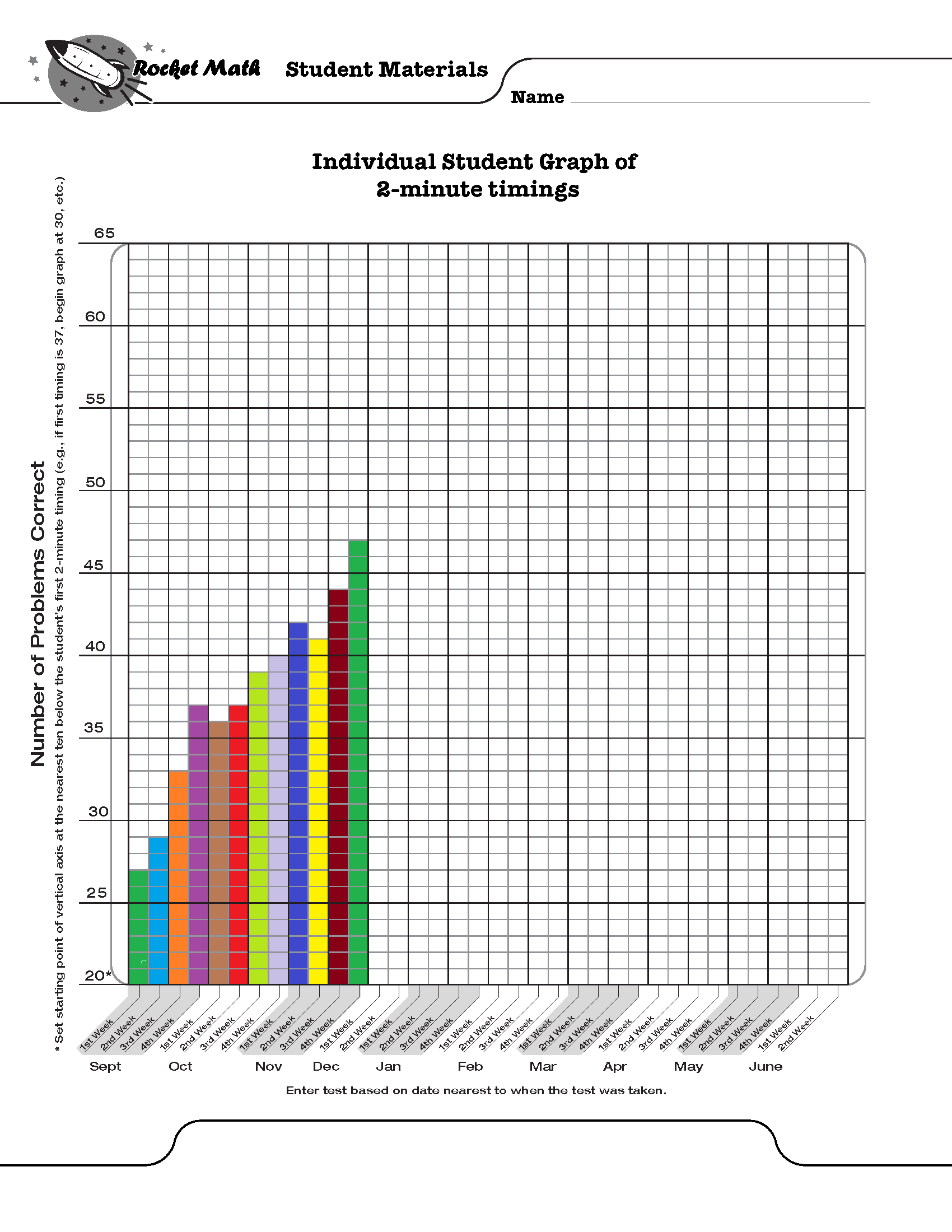Some students will need up to ten times more practice to develop math fluency than other students.  Therefore, monitoring student success in memorizing the facts is critical. Teachers can assume that what is “enough practice” for some students is NOT going to be enough practice for all students.  Effective math fluency programs must have a progress monitoring component built in.  Progress monitoring gives comparable timed tests of all the facts at intervals during the year.  Teachers look at the results of these timed tests to check on two things:

### 1. Are students gradually improving their fluency with all the facts gradually over the year?

In other words, are students able to answer more facts in the same amount of time?  If they aren’t improving, then the instructional procedures aren’t working and need to be modified or replaced.  Math fluency programs like Rocket Math’s Worksheet Program have two minute timings of all the facts in each operation that can be given and the results graphed to see if there is steady improvement.

### 2. Are all students reaching expected levels of performance at each grade level each year?

Proper math fluency programs identify students who are not meeting expectations and give them more intensive interventions.  Ultimately, by the end of fourth grade all students should be able to fluently answer basic 1s – 9s fact problems from memory in the four operations of add, subtract, multiply and divide. Fluent performance is generally assumed to be 40 problems per minute, unless students cannot write that quickly.

Expectations vary by grade level and the sequence with which schools teach facts can vary.  While it is great to achieve all that the Common Core suggests, it is critical only to assure that students master and gain fluency in 1s through 9s facts.  Some schools in some neighborhoods may find that waiting until second grade to begin math facts may not provide enough time for all students to achieve fluency.  When to begin fact fluency and how much to expect each year should be based on experience rather than some outside dictates.

## Successful math fluency programs must have these 3 features

1. ### Sequences of small sets

No one can memorize ten similar things, like the 2s facts, all at once. Students easily master math facts when they can learn and memorize small amounts of facts at one time. Effective math fluency programs define math fact sequences, which students memorize at their pace before moving onto new math facts. Rocket Math’s fluency program uses only two facts and their reverses in each set from A through Z.

2. ### Self-paced progress

Even if you only introduce small sets of math facts, some students need more time to memorize than others.  If you introduce the facts too fast, students will begin to jumble them together and progress will be lost. The pace of introducing facts must be based on mastery—not some pre-defined pace.  This is why doing all the multiplication facts as a class in the first six weeks of third grade does not work.  It is just too fast for some students.  Once they fall behind it all becomes a blur.

3. ### Effective practice and corrections

When students are practicing facts, they will come to ones they have forgotten or can’t recall immediately.  Those are the facts on which they need more practice.  Allowing students to stop and figure out the facts they don’t know while practicing, does not help the student commit them to memory.  Instead, students need to IMMEDIATELY receive the fact and the answer, repeat it and try to remember it.  Then they need to attempt that fact again in a few seconds, after doing another couple of problems.  If they have remembered the fact and can recall it, then they are on their way to fluency.  But students must practice the next day to cement in that learning.

Math fluency programs like Rocket Math’s Worksheet Program teach students math facts in small sets, allow students to progress at their own pace, and support effective practice and error correction. Each Rocket Math Worksheet program has 26 (A to Z) worksheets specially designed to help kids gradually (and successfully) master math skills. Gain access to all of them with a Universal Subscription or just the four basics (add, subtract, multiply, divide–1s to 9s) with a Basic Subscription.

## Math Teaching Strategy #1: Help students memorize math facts

### Once students know the procedure, they should stop counting and memorize!

When it comes to math facts like 9 plus 7 or 8 times 6 there are only two things to know.  1) A procedure to figure it out, which shows that you understand the “concept.”  2) What’s the answer?

It is important for students to understand the concept and to have a reliable procedure to figure out the answer to a math fact.  But there is no need for them to be required to use the laborious counting process over and over and over again!  Really, if you think about it, even though this student is doing his math “work” he is not learning anything.

## Math teaching strategy:  Go ahead and memorize those facts.

### (It won’t hurt them a bit.  They’ll like it actually.)

Once students know the procedure for figuring out a basic fact, then they should stop figuring it out and just memorize the answer.  Unlike capitals and countries in the world, math facts are never going to change.  Once you memorize that 9 plus 7 is 16, it’s good for a lifetime.  Memorizing math facts makes doing arithmetic MUCH easier and faster.  Hence our tagline

### Rocket Math: Because going fast is more fun!

Memorizing facts is the lowest level of learning.  It’s as easy as it gets.  But memorizing ALL the facts, which are similar, is kind of a long slog.  Some kids just naturally absorb the facts and memorize them.

## Math teaching strategy: Find a systematic way for students to memorize.

A lot of students don’t learn the facts and keep counting them out over and over again.  They just need a systematic way of learning the facts.  Students need to spend as much time as necessary on each small set of facts to get them fully mastered.  If the facts are introduced too fast, they start to get confused, and it all breaks down.  Each student should learn at their own pace and learn each set of facts until it is automatic–answered without hesitation and without having to think about it.  This can be accomplished by everyone, if practice is carefully and systematically set up.  It should be done, because the rest of math is either hard or easy depending on knowing those facts.  And don’t get me started about why equivalent fractions are hard!